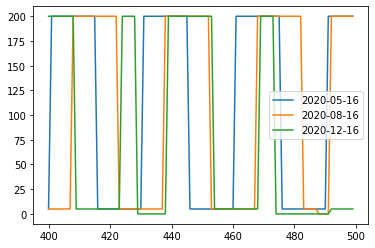# Cooking Appliances¶

In this example, appliances with multiple preferences index and attributes are modeled.

To have a better understanding of RAMP features for modelling these category of appliances, two households are considered:

1. First household with a fixed lunch habit of eating soup everyday.

2. Second household with two lunch preferences: cooking soup or rice.

The number of user preferences can be specified through “user_preference” parameter when initializing a User instance.

```# importing functions
from ramp import User,UseCase,calc_peak_time_range,yearly_pattern
import pandas as pd
```

## Creating a user category¶

```user_1 = User(
user_name = "Household with single lunch habit",
num_users = 1,
user_preference = 1, # user_1 has only one lunch preference
)

user_2 = User(
user_name = "Household with different lunch habit",
num_users = 1,
user_preference = 2, # user_2 has two lunch preferences
)
```

## Defining the cycles for cooking soup and rice¶

for cooking soup it is assumed that the user needs 25 minutes divided into two parts:

cycle

power

time

Boiling Water

1200

5

Cooking soup

750

20

for cooking rice it is assumed that the user needs 15 minutes divided into two parts:

cycle

power

time

Boiling Water

1200

5

Cooking rice

600

10

```# soup for lunch
name = "soup for lunch",
power = 1200,
func_time = 25,
func_cycle = 25,
thermal_p_var = 0.2,
fixed_cycle = 1,
window_1 = [12*60,15*60],
p_11 = 1200,   # power of the first cycle
t_11 = 5,      # time needed for the first cycle
p_12 = 750,    # power of the second cycle
t_12 = 20,     # time needed for the second cycle
cw11 = [12*60,15*60]
)
```

As second user has two different preferences for lunch, we need to model this preferences and their characterisitics as two different appliances.

Each preference needs to be specified with the cooking energy needs like the power, functioning time and the duty cycles of the cooking process.

More importantly, for each preference, the user needs to specify the index of preference by using pref_index parameter. In this example, soup is the first prefernce of the user (pref_index = 1), and rice is the second one (pref_index = 2).

```# soup for lunch
name = "soup for lunch",
power = 1200,
func_time = 25,
func_cycle = 25,
thermal_p_var = 0.2,
fixed_cycle = 1,
pref_index = 1, # the first preference
window_1 = [12*60,15*60],
p_11 = 1200,   # power of the first cycle
t_11 = 5,      # time needed for the first cycle
p_12 = 750,    # power of the second cycle
t_12 = 20,     # time needed for the second cycle
cw11 = [12*60,15*60]
)
```
```# rice for lunch
name = "rice for lunch",
power = 1200,
func_time = 15,
func_cycle = 15,
thermal_p_var = 0.2,
pref_index = 2,  # the second preference
fixed_cycle = 1,
window_1 = [12*60,15*60],
p_11 = 1200,   # power of the first cycle
t_11 = 5,      # time needed for the first cycle
p_12 = 600,    # power of the second cycle
t_12 = 10,     # time needed for the second cycle
cw11 = [12*60,15*60]

)
```
```# you can have an overview of data inputs by usering User.export_to_dataframe method
user_lunch = UseCase(users=[user_1,user_2])
user_lunch.export_to_dataframe().T
```
0 1 2
user_name Household with single lunch habit Household with different lunch habit Household with different lunch habit
num_users 1 1 1
user_preference 1 2 2
name soup for lunch soup for lunch rice for lunch
number 1 1 1
power 1200.0 1200.0 1200.0
num_windows 1 1 1
func_time 25 25 15
time_fraction_random_variability 0 0 0
func_cycle 25 25 15
fixed no no no
fixed_cycle 1 1 1
occasional_use 1 1 1
flat no no no
thermal_p_var 0.2 0.2 0.2
pref_index 0 1 2
wd_we_type 2 2 2
p_11 1200 1200 1200
t_11 5 5 5
cw11_start 720 720 720
cw11_end 900 900 900
p_12 750 750 600
t_12 20 20 10
cw12_start 0 0 0
cw12_end 0 0 0
r_c1 0 0 0
p_21 0 0 0
t_21 0 0 0
cw21_start 0 0 0
cw21_end 0 0 0
p_22 0 0 0
t_22 0 0 0
cw22_start 0 0 0
cw22_end 0 0 0
r_c2 0 0 0
p_31 0 0 0
t_31 0 0 0
cw31_start 0 0 0
cw31_end 0 0 0
p_32 0 0 0
t_32 0 0 0
cw32_start 0 0 0
cw32_end 0 0 0
r_c3 0 0 0
window_1_start 720 720 720
window_1_end 900 900 900
window_2_start 0 0 0
window_2_end 0 0 0
window_3_start 0 0 0
window_3_end 0 0 0
random_var_w 0 0 0

## Generating a profile for some months¶

```peak_time_range = calc_peak_time_range(
user_list = user_lunch.users
)
year_behaviour = yearly_pattern()
```
```# number of days
n_days = 90

# storing all the profiles for all the users
profiles = pd.DataFrame(index = pd.date_range(start = "2020-01-01",periods = 1440*n_days,freq="T"))

for user in user_lunch.users:

# storing daily profiles for a user
user_profiles = []
for day in range(n_days):
prof_i = day, # the day to generate the profile
peak_time_range = peak_time_range,
Year_behaviour = year_behaviour
)

user_profiles.extend(single_profile)

profiles[user.user_name] = user_profiles
```

As the second user has the probability of cooking rice for lunch, and lunch has a less energy intensive cooking cycle, it is expected to see that the user with fixed lunch meal has higher energy consumption in most of the cases.

```# daily energy consumption
profiles.resample("1d").sum().plot(title = "daily energy consumption")
```
```<AxesSubplot:title={'center':'daily energy consumption'}>
```